# Maximum value of induced EMF

## Homework Statement

In the very long wire conductor as shown on the picture , flows a current magnitude I. Near the wire there is a
square shaped contour , with lenght a ,which rotates around its axis a constant angular velocity. Axis of square contour and a straight line conductor are parallel , and the distance between them is b = 3*a / 2 .
Environment is the vacuum. Find the expression for the maximum value of induced EMF e(t) induced in the contour. Ignore the self-inductance emf.
Solution e max = 3*u (zero)* I * w * a / 10*pi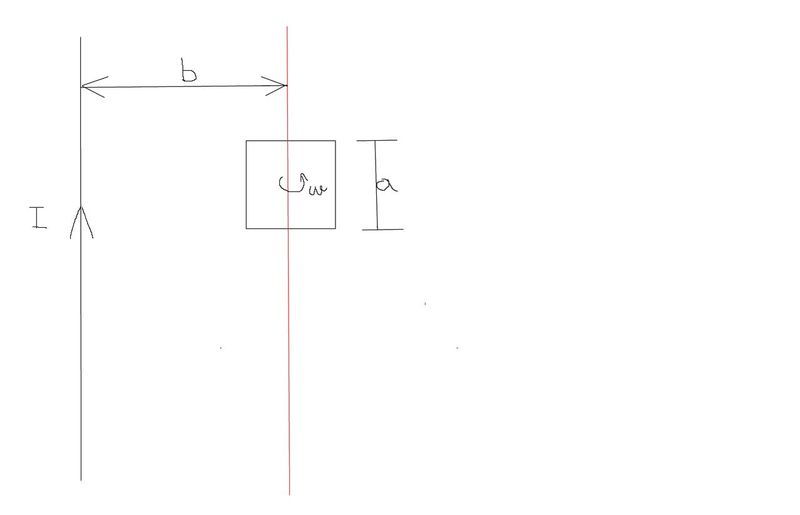## The Attempt at a Solution

I can't find relation between theta 1 and angle fi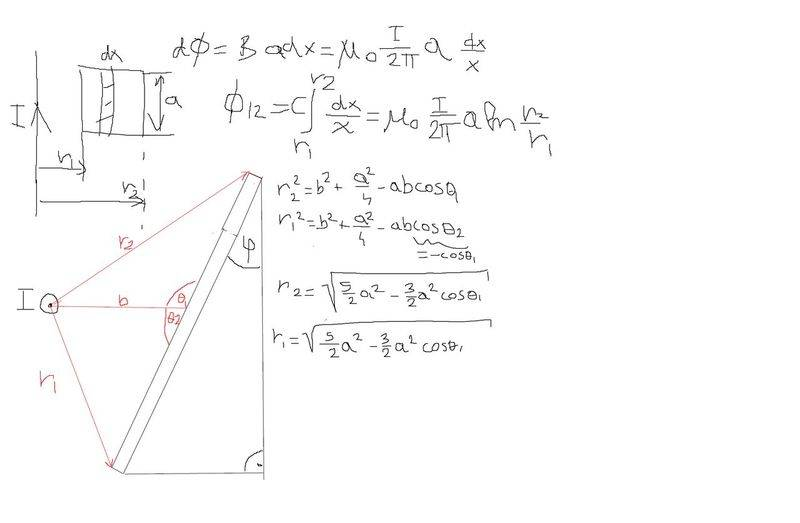I can't find relation between theta 1 and angle fi

well i could not understand the diagram involving those angles - as your loop is rectangular and its arms are either parallel to current flow or perpendicular
B -you can write using the field due to a long(infinite) current carraying conductor-wire and flux you are calculating which is being swept by the loop
so one can calculate induced e.m.f. from the rate of change of flux.
in your diagram -what is that strip doing?

haruspex
Homework Helper
Gold Member
well i could not understand the diagram involving those angles - as your loop is rectangular and its arms are either parallel to current flow or perpendicular
Yes, but it is rotating. The diagram is a view along the axis of rotation at an arbitrary time.
@Ivan Antunovic , in your diagram, draw a vertical line through the middle of the frame. What parallel lines do you see?

Yes, but it is rotating. The diagram is a view along the axis of rotation at an arbitrary time.
@Ivan Antunovic , in your diagram, draw a vertical line through the middle of the frame. What parallel lines do you see?
Yes you are right it's rotating , @drvrm I am sorry if my diagram was bad , I tried my best.

@haruspex I drew vertical line and I see two triangles that are blue triangle 1 and blue triangle 2 similiar and that they have both the same angles.If that was your point?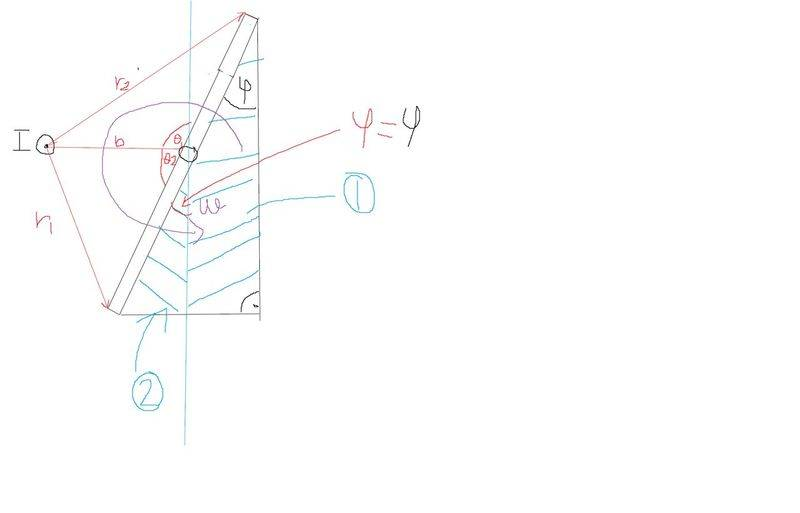free photo hosting

Hope that is what you meant.

Also I tried my calculations with angle theta 2 for magnetic flux and induced emf as a function of time (theta2 = wt) and I got: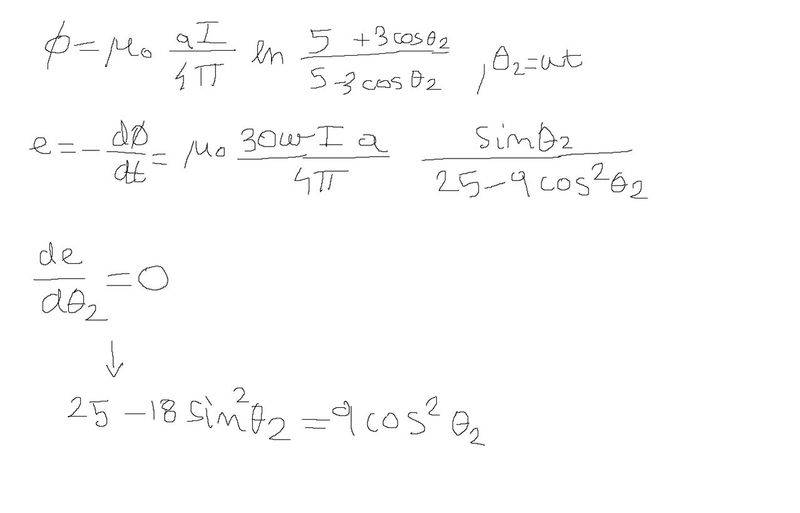tried to take derivative e(t) in respect to theta2 to get angle for maximum of function e(t) and I got:

-18(tan(x))^2 = 9 - (25/(cosx)^2)

which gives imaginary solutions.

tried to take derivative e(t) in respect to theta2 to get angle for maximum of function e(t) and I got:
-18(tan(x))^2 = 9 - (25/(cosx)^2)

which gives imaginary solutions.

i have a hunch that the rectangular frame is rotating about an axis parallel to the conducting wire(Through which the current is flowing)
and the frames vertical arms will rotate to form a cylindrical sweep about the axis witha diameter equak to width of the rectangular frame.
therefore the arms of the frame will be parallel and the width will be perpendicular to the conducting wire(so no question of angular variations arise);try to imagine the flux passing through the area of the loop by integrating B.dA where dA is an elementary area
and then using Flux and angual velocity you can get the induced e.m.f.
if you need further help i can provide you some net-source which may /may not help you.

haruspex
Homework Helper
Gold Member
Your 5/2 factors inside the square roots are wrong.
I don't know where your equation with a ln term in it comes from. I'm not that familiar with electromagnetics. Can you post a link as reference?
It seems intuitive that the maximum would be at Φ=0.

i have a hunch that the rectangular frame is rotating about an axis parallel to the conducting wire(Through which the current is flowing)

Correct, you got it .

therefore the arms of the frame will be parallel and the width will be perpendicular to the conducting wire(so no question of angular variations arise);try to imagine the flux passing through the area of the loop by integrating B.dA where dA is an elementary area
and then using Flux and angual velocity you can get the induced e.m.f.
if you need further help i can provide you some net-source which may /may not help you.

Well you can see it from my calculations I was doing the same thing just integrating by imagionary loop.
I found dA element(dS) and integrated it over r1 to r2, then I found flux as a function of time and took derivative of magnetic flux and that is fine , I get same results as in solutions, but their angle for maximum emf is pi/12 and therefore final solution is emax= 3*u0*I*w*a/10*pi
So you meant imagining loop this way as shown on my picture?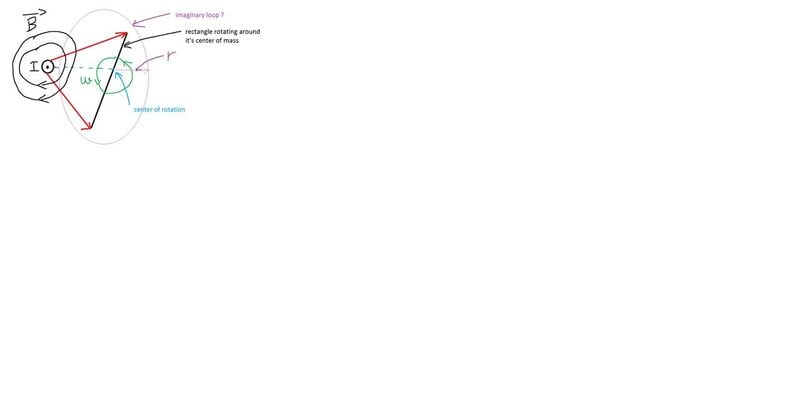And of course I am always happy if I can get free source that can help me.

Your 5/2 factors inside the square roots are wrong.
I don't know where your equation with a ln term in it comes from. I'm not that familiar with electromagnetics. Can you post a link as reference?
It seems intuitive that the maximum would be at Φ=0.

The problem is that solution should be in analyitical form, their solutions are giving the same result for magnetic flux Φ so I doubt it's wrong.
http://physics.ucsd.edu/students/courses/winter2008/managed/physics1bc/documents/Ch20notesforweb.pdf [Broken]

Maximum induced emf will be when Φ is maximum , so it's when vector dA and vector B are making cos(180) and cos(0) ,since B*dA*cos(B,dA(vectorially))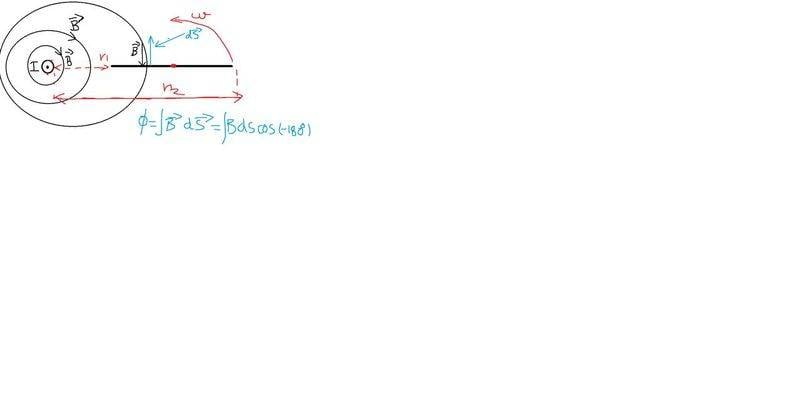windows print screen

Last edited by a moderator:
haruspex
Homework Helper
Gold Member
2
Maximum induced emf will be when Φ is maximum , so it's when vector dA and vector B are making cos(180) and cos(0) ,since B*dA*cos(B,dA(vectorially))
Well, it's not quite trivial because this is not a uniform field. The flux is denser closer to the wire. Also, when the square frame is at the φ=0 position, the sides of it are not travelling perpendicular to the field.
But you have not responded to my remark that the 5/2 factors are wrong in your expressions for r1 and r2 in your post #1.

2
Well, it's not quite trivial because this is not a uniform field. The flux is denser closer to the wire. Also, when the square frame is at the φ=0 position, the sides of it are not travelling perpendicular to the field.
But you have not responded to my remark that the 5/2 factors are wrong in your expressions for r1 and r2 in your post #1.

Well , for me it's most intuitive that most flux will be flowing through rectangle when the position of the rectangle is at closest position to the wire .

I don't see how 5/2 factors are wrong
since
r^2 = b^2 + 1/4 * a^2 - ab cos(theta1) , and b = 3*a / 2 ,

so we get r^2 = 9/4 * a^2 + 1/4 * a^2 - a^2 * 3/2 * cos(theta1) = 5/2 * a^2 - 3/2 * a^2 * cos(theta1) ,
that is what I am getting maybe I am wrong

And of course I am always happy if I can get free source that can help me.

<
web.mit.edu/viz/EM/visualizations/coursenotes/modules/guide10.pdf>

TSny
Homework Helper
Gold Member
Maximum induced emf will be when Φ is maximum

The induced emf is not proportional to Φ, it's proportional to the rate of change of Φ.

Your result for Φ looks correct. You just need to find the maximum value of dΦ/dt.

haruspex
Homework Helper
Gold Member
I don't see how 5/2 factors are wrong
since
sorry, my mistake.

haruspex
Homework Helper
Gold Member
most intuitive that most flux will be flowing through rectangle when the position of the rectangle is at closest position to the wire
As TSny points out, the emf depends on the rate at which the wire cuts field lines. When one side is closest to the wire, the sides of the square are travelling parallel to the field lines.
It's not obvious when one side of the square will cut field lines at its greatest rate. It won't be when it is travelling perpendicularly to the field lines, but a little closer to the wire than that, since closer to the wire the field lines are denser. But you don't need it maximised for one side of the square, you need it maximised for the square as a whole, and it is intuitive that that will be when the two sides of the square are at the same distance from the wire.

Maybe I expressed myself incorrectly , of course e induced depends on the greatest change , just the max induced emf will be when the plate is at angle = 0 , or as @haruspex said : when one side is closest to the wire

Double post , apologies.

The induced emf is not proportional to Φ, it's proportional to the rate of change of Φ.

Your result for Φ looks correct. You just need to find the maximum value of dΦ/dt.

For dFi / dt = e

e(t) = ( ( u0 * I * 30 * w * a )/ (4*pi) )* sin(wt)/(9- 25 cos^2(wt) )

but since e(t) = emax * sinwt+fi I need to make my my sin(wt)/(9- 25 cos^2(wt) )= sin(wt+fi)

Last edited:
TSny
Homework Helper
Gold Member
For dFi / dt = e

e(t) = ( ( u0 * I * 30 * w * a )/ (4*pi) )* sin(wt)/(9- 25 cos^2(wt) )
OK, except I get an expression where the 9 and 25 in the denominator switch places.

but since e(t) = emax * sinwt+fi I need to make my my sin(wt)/(9- 25 cos^2(wt) )= sin(wt+fi)
The emf in this problem will not vary as ##\sin( \omega t +\phi)## due to the fact that the B field is not uniform.

OK, except I get an expression where the 9 and 25 in the denominator switch places.

The emf in this problem will not vary as ##\sin( \omega t +\phi)## due to the fact that the B field is not uniform.
Yes you are right, I forgot about that. Tried to take derivative of de(theta)/dtheta and making it equal to 0 ,for getting the maximum out of my function e(t)

de/d(theta) = 25 -9 cos^2(theta) - 18sin^2(theta) = 0

And I really don't know how to calculate this .

9( -cos^2(theta) - 2 sin^2(theta) ) + 25 =0

it's simple math but seems like I wasn't doing calculations of this type so long

Last edited:
TSny
Homework Helper
Gold Member
de/d(theta) = 25 -9 cos^2(theta) - 18sin^2(theta) = 0
Did you also have an overall factor of cosθ? Can you use that to make de/dθ = 0?

(Note that there is a formatting tool bar. It has superscript and subscript formatting. If you click on the Σ symbol, you can see some math symbols.)

9( -cos^2(theta) - 2 sin^2(theta) ) + 25 =0

it's simple math but seems like I wasn't doing calculations of this type so long
Using cos2θ = 1-sin2θ you should be able so see that this has no solution. That's why that cosine factor is important.

Did you also have an overall factor of cosθ? Can you use that to make de/dθ = 0?

(Note that there is a formatting tool bar. It has superscript and subscript formatting. If you click on the Σ symbol, you can see some math symbols.)

Using cos2θ = 1-sin2θ you should be able so see that this has no solution. That's why that cosine factor is important.
Sorry for the lack of understanding of some english terms, what do you mean when you say an overall factor of cosθ ?

TSny
Homework Helper
Gold Member
Sorry for the lack of understanding of some english terms, what do you mean when you say an overall factor of cosθ ?
Did you get

de/dθ ∝ cos(θ)[25 -9 cos2θ - 18sin2θ]

Did you get

de/dθ ∝ cos(θ)[25 -9 cos2θ - 18sin2θ]
I got:
9 * cos^2θ - 25 + 18 * sin^2θ - 25 = 0

TSny
Homework Helper
Gold Member
When you calculated de/dθ you should have gotten de/dθ = Acos(θ)[25 -9 cos2θ - 18sin2θ]/(some denominator), where A is a constant factor and I have not bothered to write out the expression for the denomintor.

When you calculated de/dθ you should have gotten de/dθ = Acos(θ)[25 -9 cos2θ - 18sin2θ]/(some denominator), where A is a constant factor and I have not bothered to write out the expression for the denomintor.

So it should be 25 - 9 *( 1 - sin^2θ) -18sin^2θ =0 ?

I get from that
sin^2θ = 4/ sqrt(27)
θ = 50.34 ° ,
Solution is θ = 90°

TSny
Homework Helper
Gold Member
So it should be 25 - 9 *( 1 - sin^2θ) -18sin^2θ =0 ?
What happened to the cosine factor in red? cos(θ)[25 -9 cos2θ - 18sin2θ]

I get from that
sin^2θ = 4/ sqrt(27)

I think you made a mistake with some signs. The equation 25 -9 cos2θ - 18sin2θ = 0 has no real solution for θ.

What happened to the cosine factor in red? cos(θ)[25 -9 cos2θ - 18sin2θ]

I think you made a mistake with some signs. The equation 25 -9 cos2θ - 18sin2θ = 0 has no real solution for θ.

since cos(θ)[25 -9 cos2θ - 18sin2θ] = 0 I divided both sides with cos(θ), well I don't know why aren't we getting solutions in real domain.

haruspex
Homework Helper
Gold Member
Tried it now in wolfram alpha

http://postimg.org/image/ufv9m9rcv/f9cb9c53/

θ = π/ 2 , for n = 1

I don't get it why are we getting wrong solutions
If you divide out by a factor, you need to consider whether that was a valid division. What must you never divide by?

•Ivan Antunovic
TSny
Homework Helper
Gold Member
since cos(θ)[25 -9 cos2θ - 18sin2θ] = 0 I divided both sides with cos(θ), well I don't know why aren't we getting solutions in real domain.
When you divided through by cos(θ), you lost the solution. (This is a type of mistake I've made more than once)

•Ivan Antunovic
If you divide out by a factor, you need to consider whether that was a valid division. What must you never divide by?
By zero of course , so it should be cos(θ) = 0 , which gives θ =π / 2.

Thank you guys for your help and your times ,I really appreciate it , you helped big time!

Last edited:
I have one more question , suppose we weren't using derivatives to find our angle θ for maximum emf induced, because it takes too much time ,
when θ = 0 and since cosθ , the magnetic flux will be maximum , emf induced is derivative of magnetic flux so it is connected with sinθ and sinθ is maximum for π/2.

I think that Force should be in the direction as shown on the picture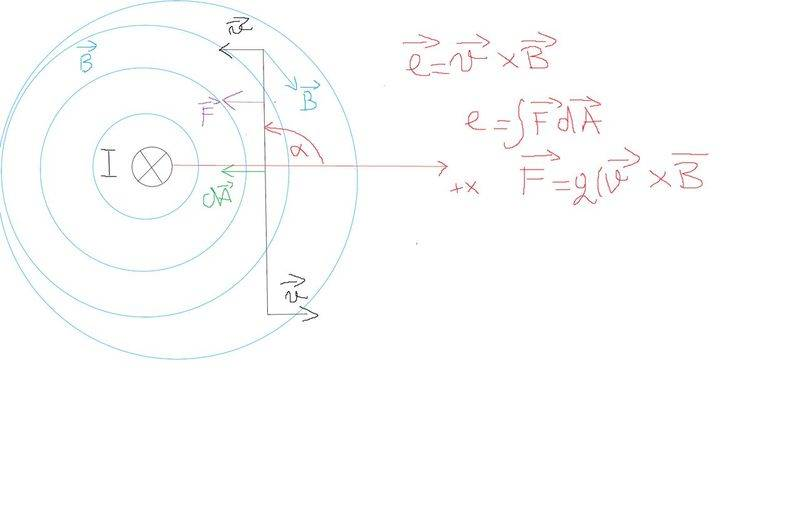image sharing

by definition
F = q(v x B), and e = integral ( (vxB) * dA) )
I can't find cross product of v x B with right hand rule since they are not making angle of 90 degrees,
and I should use matrix calculations to find it but that again involves a lot of math ,
but both F and dA should make angle of 0 degrees to get our maximum emf induced,
so that we get cos(0) = 1 , and therefore e = F*A *cos(0) = F*A , where we get our maximum induced emf.
Is my thinking legit?

Last edited:
TSny
Homework Helper
Gold Member
I think that Force should be in the direction as shown on the picture
The cross product v x B produces a vector that is perpendicular to the plane containing v and B.

F = q(v x B), and e = integral ( (vxB) * dA) )
The integral should not be an integral over a surface. It should be an integral along a line.
I can't find cross product of v x B with right hand rule since they are not making angle of 90 degrees,
and I should use matrix calculations to find it but that again involves a lot of math
You'll need to decide which sides of the square contribute to the emf. Once you do that, it will not be too difficult to find the angle between v and B for the sides that contribute. You will not need to use a matrix to find the cross product.
but both F and dA should make angle of 0 degrees to get our maximum emf induced,
so that we get cos(0) = 1 , and therefore e = F*A *cos(0) = F*A , where we get our maximum induced emf.
Is my thinking legit?

Force times area does not have the dimensions of emf. Area will not be relevant here.

Edit: Also, it might make the geometry a little easier to have the current coming out of the page in your previous picture. Then the angle between v and B will be less than 90o.

The cross product v x B produces a vector that is perpendicular to the plane containing v and B.

The integral should not be an integral over a surface. It should be an integral along a line.

You'll need to decide which sides of the square contribute to the emf. Once you do that, it will not be too difficult to find the angle between v and B for the sides that contribute. You will not need to use a matrix to find the cross product.

Force times area does not have the dimensions of emf. Area will not be relevant here.

Edit: Also, it might make the geometry a little easier to have the current coming out of the page in your previous picture. Then the angle between v and B will be less than 90o.

Sorry for the late replay.
it's e = 1 /q closed loop integral ( F dS), I tried to draw some positions of my contour and as my angle goes from 90 - 180, angle between v and B becomes greater and then their vector product becomes less.After 180 degrees emf gets negative and becomes minimum at angle -270 degrees , that is what I see from my drawings. Also the force is coming out of the board as you said? Well I am so stupid because of thinking that force should be in the direction of v.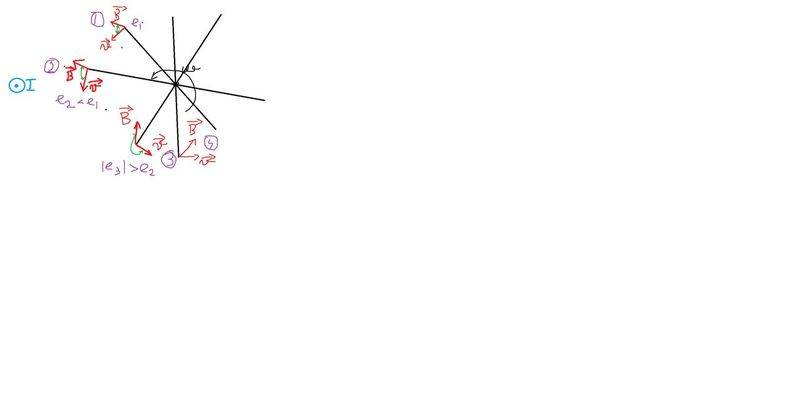image hosting no register

TSny
Homework Helper
Gold Member
You will need to consider each of the 4 sides of the loop separately. For example, the figure below shows looking down on the top side of the loop, similar to your picture. The green dots show arbitrary points on the top side. As you see, the direction of v x B is out of the page or into the page. Therefore, is any emf developed along this side?

Next consider one of the sides of the loop that is parallel to the current.

#### Attachments

Last edited:
•Ivan Antunovic
You will need to consider each of the 4 sides of the loop separately. For example, the figure below shows looking down on the top side of the loop, similar to your picture. The green dots show arbitrary points on the top side. As you see, the direction of v x B is out of the page or into the page. Therefore, is any emf developed along this side?

Next consider one of the sides of the loop that is parallel to the current.

If I am right ,at the top green dot the direction of v x B direction is into the board , and at the bottom green line the v x B product is out of the board , and the angle between v x B is the same both on top and bottom green dot , therefore emf of the same magnitude will be produced just opposite direction ?